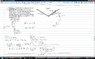# Block inside a spinning cone - Newton's 2nd Law problem

• Bensky
so, the weight is always acting along the vertical direction and the normal force is acting along the perpendicular direction.

#### Bensky

Block inside a spinning cone -- Newton's 2nd Law problem

## Homework Statement

See attempt at solution. I have attached everything there.

## Homework Equations

F_net = m * a_n (net force)
W = mg (weight)
a_n = 4*pi^2 * R * f^2 ... formula for "normal" component of acceleration where R = radius, f = rotating frequency

## The Attempt at a Solution

Some questions I have about this problem (look at my work below first or these might not make any sense) --
1. Why is there only a vertical component for the weight? I thought weight always has 2 components whenever an object is on an "incline" which it appears to be here. Does this have something to do with the motion being circular?

2. Why does the normal force have two components if the weight does not? (Ncos(theta) and Nsin(theta))? And further, when exactly do you calculate the normal force or weight using components and when do you not? For example I know on all the incline plane problems I've done, the weight always has two components but normal force is just calculated as N - not using NSin(theta) or NCos(theta).

Basically, in my attempt below, I was just following the method my professor used to solve a similar problem (only difference was his problem had a sphere instead of a block and there was no friction) and I don't really understand why he calculated two above quantities the way he did. I did not get the correct answer so I'm trying to figure this out and having a hard time.

So here's my work:

#### Attachments

•attempt3.jpg
52 KB · Views: 1,196

Friction acts down the slope - this is where you messed up. That means
N sin(theta) + (mu)*N*cos(theta) = m*a
along the radius of the circular path.

Regarding weight's components . Components always along different directions regardless whether the body's on flat ground or a slope. Here your analysis is along the direction of gravity and normal to it - so there is no m*g in one eqn. and complete m*g in other. By the same token normal force has two components as it has some magnitude along both axes we analyse. Remember components are made for our convenience - it isn't that they actually exist uniquely. If I had chosen to analyse along different axes - say one down the plane and other perpendicular to it (which in fact I had) I would have had components of weight and not of the normal force.

aim1732 said:
Friction acts down the slope - this is where you messed up. That means
N sin(theta) + (mu)*N*cos(theta) = m*a
along the radius of the circular path.

Regarding weight's components . Components always along different directions regardless whether the body's on flat ground or a slope. Here your analysis is along the direction of gravity and normal to it - so there is no m*g in one eqn. and complete m*g in other. By the same token normal force has two components as it has some magnitude along both axes we analyse. Remember components are made for our convenience - it isn't that they actually exist uniquely. If I had chosen to analyse along different axes - say one down the plane and other perpendicular to it (which in fact I had) I would have had components of weight and not of the normal force.
I sort of understand your explanation. I'll have to read over it again, but thanks.

Btw - If I use N*sin(theta) + mu * N * cos(theta) I get frequency to be equal to .788, which is still not correct.

Here is a picture of the updated work:

#### Attachments

You should also have a component of the friction in the y-plane.

benhou said:
You should also have a component of the friction in the y-plane.
Thank you, I don't know why I didn't see that. I now have the correct answer.

I'm still a little shaky on when you use components for certain things even after reading over aim's explanation again. Could someone else try explaining this in a different way if possible?

Bensky said:
Thank you, I don't know why I didn't see that. I now have the correct answer.

I'm still a little shaky on when you use components for certain things even after reading over aim's explanation again. Could someone else try explaining this in a different way if possible?

In this particular problem, we have the object accelerating towards the centre. It implies that the net force is towards the centre. If we were to separate the forces into components, one of which is in the direction of the net force, it would be more convenient, because one of them will yield a zero, the other will just be the net force. In this case, $$F_{y}=0 , F_{x}=ma_{c}$$

Hope it helps.